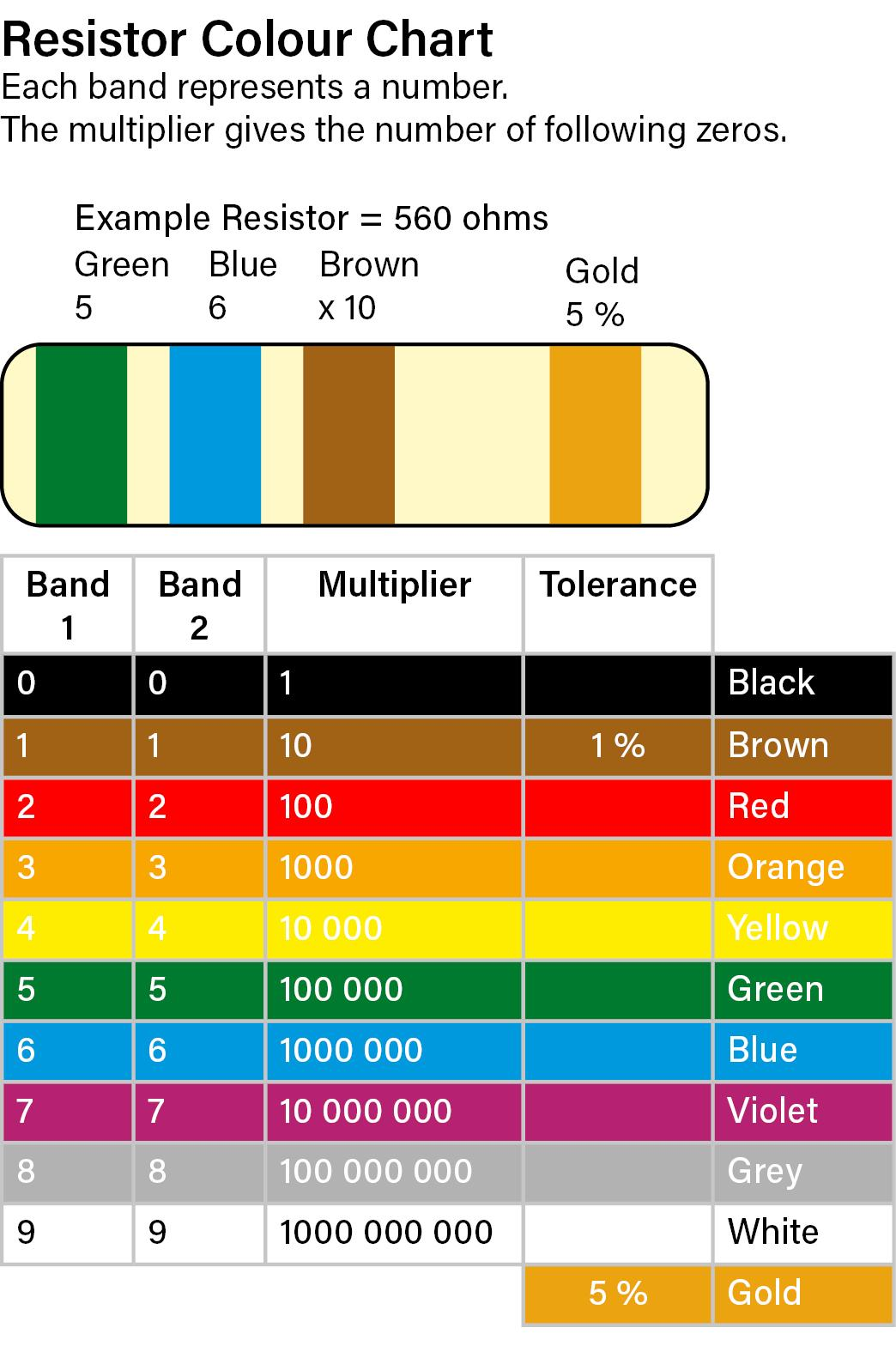# Resistor Guide - Resistor Colour Bands and Schematic Lables

We sell:

Carbon Film Resistors with 5% tolerance at 1/4w / 0.25w

Metal Film Resistors with 1% tolerance at  1/4w / 0.25w

You can work out the resistance by looking at the coloured bands.
The most common resistors have 4 or 5 bands.

More information on this and a colour chart further down the page

How to read or write Resistance on a Schematic or Label
Resistance is measured in ohms and there are some standard ways of writing this.
Single figures can either be given the symbol for ohm: Ω or just R
So a 10 ohm resistor can be 10Ω or 10R

• At 1000 ohms or more the symbol K is used (for kilohms);
So a 1000 ohm resistor is usually written as 1K
• A 'between' value such as 4700 ohms can be written as 4.7K or as 4K7
• Above 10K the K symbol is the standard until you reach one million ohms and the symbol M (for megohms);
so a 1 Million ohm resistor is written as 1M or 1MΩ

Scroll down for our handy colour chart

The first two or three bands give number values and the third or fourth band gives the number of zeros
(or how many times 10 to multiply the number by).

The last band (usually slightly separated) gives the tolerance:
for example a 100 ohm resistor at 5% will be between 95 and 105 ohms but a 100 ohm resistor at 1% will be between 99 and 101 ohms.
If you test some with a meter, you will find that they are actually remarkably accurate - it's unusual to find a resistor these days that is far from its stated value.

Note that Black can be 0 in a number band or it can mean multiply by 1x in the multiplier band.

For example:
1 ohm resistor: Black (0) Brown (1) Black (x1)
10 ohm resistor: Brown (1) Black (0) Black (x1)
100 ohm resistor: Brown (1) Black 0) Brown (x10)

We'll send you a printed copy of this handy chart to keep: